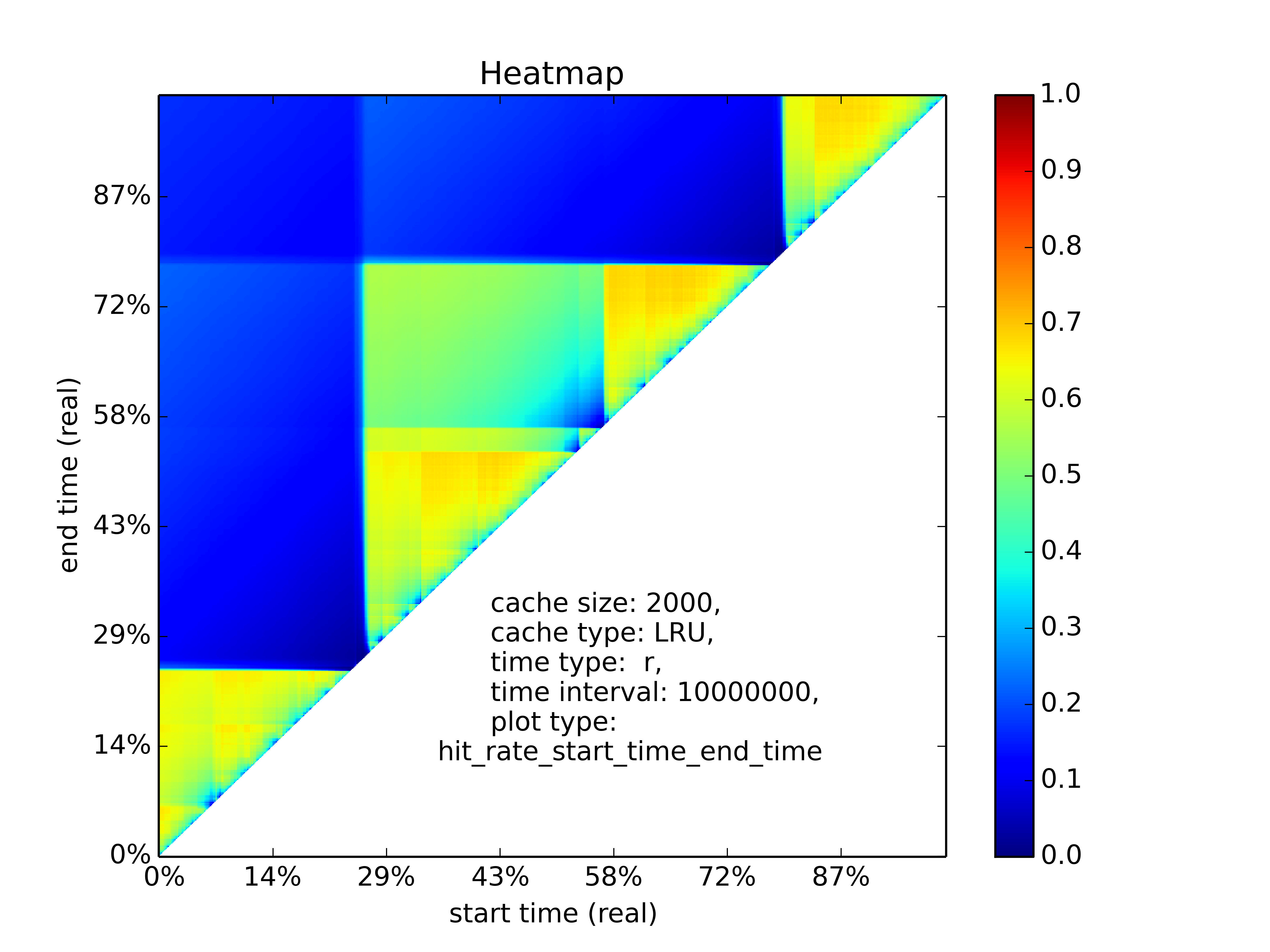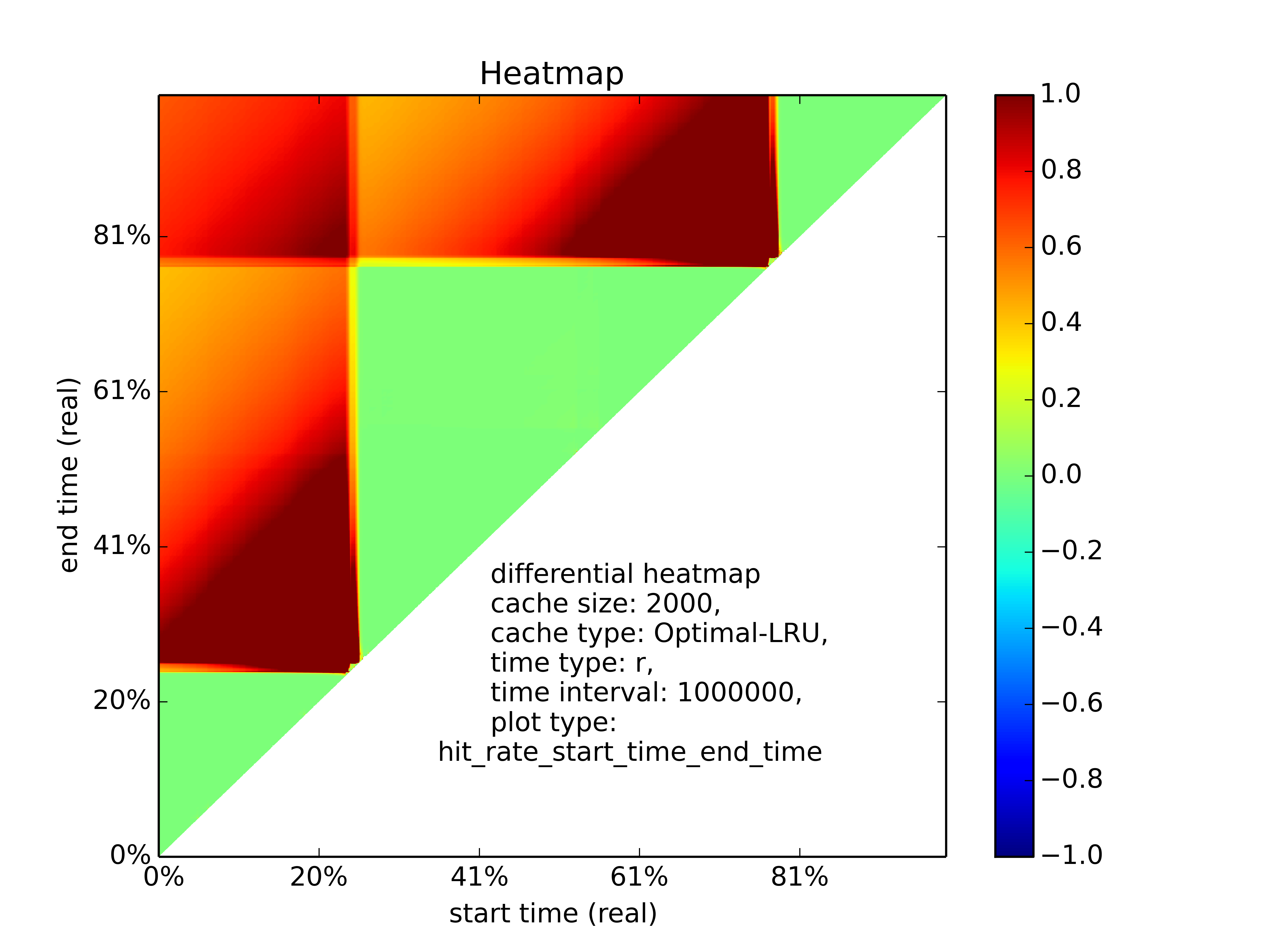# Heatmap Plotting¶

## Plotting Heatmaps¶

Another great feature of PyMimircache is that it allows you to incorporate time component into consideration, taking cache analysis from static to dynamic. Currently six types of heatmaps are supported.

### Plot Types¶

For all the plot types, the X-axis is the time in percent (either real or virtual).

 plot type Y-axis plot detail hit_ratio_start_time_end_time end time (real or virtual) in percent pixel (x, y) means the hit ratio from time x to time y. rd_distribution reuse distance reuse distance distribution graph, pixel (x, y) represents at time x+time_interval, the number of requests have reuse distance of y (shown in color). rd_distribution_CDF reuse distance similar to reuse distance distribution graph, but each points (x, y) represents the percent of requests have reuse distance less than or equal to y. future_rd_distribution future reuse distance future reuse distance distribution graph, future reuse distance is defined as how far in the future, it will be accessed again. hit_ratio_start_time_cache_size cache size each vertical line x=t is a hit ratio curve of trace starting at t avg_rd_start_time_end_time end time (real or virtual) in percent pixel (x, y) means average reuse distance of requests from time x to time y

### How to Plot¶

Plotting heatmaps is easy, just call the following function on cachecow,

```>>> c.heatmap(time_mode, plot_type, time_interval=-1, num_of_pixels=-1,
algorithm="LRU", cache_params=None, cache_size=-1, **kwargs)
```

The first two parameters are mode and plot_type, time mode is either r or v for real time or virtual time, the types of plot(see table above) The following keyword arguments are optional, however, you must provide one of time_interval and number_of_pixels, which controls how fine the graph will look and also determines the amount of computation. The time_interval variable implies the time span of a single pixel in the plot, sometimes it is not easy to estimate time_interval, so instead you can provide the number of pixels you want in one dimension.

Note

If you do not want the computation time to be very long, then specify a big time_interval or small num_of_pixels.

 Keyword Arguments Default Value Possible Values Necessary time_interval “-1” a time interval provide this value or num_of_pixels num_of_pixels “-1” the number of pixels on one dimension provide this value or time_interval algorithm “LRU” All available cache replacement algorithms No cache_params None Depends on cache replacement algorithms Depends on cache replacement algorithms, for example LRU_K cache_size -1 Positive integer Necessary for plot “hit_ratio_start_time_end_time” figname heatmap.png Any string, remember to include suffix No num_of_threads 4 Positive integer except 0 No

Attention: cache_size is necessary for hit_ratio_start_time_end_time graph.

### Plotting Examples¶

```>>> c.heatmap('r', "hit_ratio_start_time_end_time", num_of_pixels=200, cache_size=2000, figname="heatmap1.png", num_of_threads=8)
```Hit ratio of varying start time and end time

Another example

```>>> c.heatmap('r', "rd_distribution", time_interval=10000000)
```

## Plotting Differential Heatmaps¶

Want to know which algorithm is better? Not satisfied with hit ratio curve or miss ratio curve because they only show you the result over the whole trace? You are in the right place! Differential heatmaps allow you to compare cache replacement algorithms with respect to time.

Currently we only support differential heatmap of hit_ratio_start_time_end_time. Each pixel (x, y) in the plot represents the hit ratio difference between algorithm1 and algorithm2 divided by hit ratio of algorithm 1 from time x to y. The function to plot is shown below:

```>>> c.diff_heatmap(time_mode, plot_type, algorithm1, time_interval=-1, num_of_pixels=-1,
algorithm2="Optimal", cache_params1=None, cache_params2=None, cache_size=-1, **kwargs)
```

The arguments here are similar to plotting heatmaps, the only difference is that we have one more algorithm, which is used for comparison,

Example:

```>>> c.diff_heatmap('r', "hit_ratio_start_time_end_time", time_interval=1000000, algorithm1="LRU", cache_size=2000)
```Differential heatmap, the value of each pixel is (hit_ratio_of_algorithm2 - hit_ratio_of_algorithm1)/hit_ratio_of_algorithm1

Congratulations! You have finished the basic tutorial! Check Advanced Usage Part if you need.